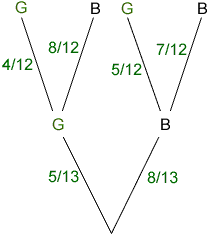Quandaries and Queries I am sending this for a student because she does not have the Internet. These two problems stumped us and we are going crazy. A bowl contains five green olives and eight black ones. Wilma sticks a fork into a bowl and brings out two olives. What is the probability that she gets one green and one black olive? A. 1/2 B. 10/39 C.40/169 D. 5/8 What is the probability that there are exactly two girls in a family of three children? A. 2/3 B.3/8 C.1/2 D.1/4 Thank you very much for your time. annetta Hi Annetta, I'll do the first one and then you can tell me if it helps you with the second. I would approach both these problems with a tree diagram. For the first problem think of selecting one olive from the jar and then a second. Draw a tree which represents this two step process and write the appropriate probability beside each branch of the tree.In the diagram G represents a green olive and B a black olive.Start at the bottom of the tree. There are 5 green olives and 8 black olives so when you stick the first olive the probability that it is green is 5/13 and the probability that it is black is 8/13. Hence I wrote these probabilities beside the bottom two branches of the tree. If you got a green olive then you have climbed the left branch of the tree and the jar has 4 green and 8 black remaining. Stick a second olive. The probability it is green is 4/12 and the probability that it is black is 8/12. Thus I labeled the second level of branches, on the left of the tree, with these probabilities. In a similar fashion, if you got a black olive the first time then there are 5 green and 7 black remaining. Hence the probabilities on the right side of the tree. If you have a green olive and a black olive then you got them in one of two ways: a green olive and then a black olive this has probability 5/138/12 or a black olive and then a green olive this has probability 8/135/12 The probability that you have a green olive and a black olive is the probability that you selected a green olive and then a black olive or a black olive and then a green olive. This has probability ( 5/138/12) + (8/135/12) = 20/39 Harley Go to Math Central